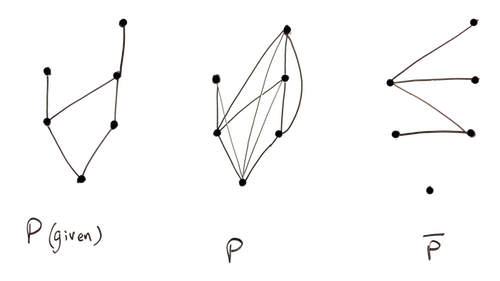# Graph Entropy, Posets, and Sorting Under Partial Information

### Graph Entropy, Posets, and their applications in SUPI (pdf)

Preetum Nakkiran and Matthew Francis-Landau. 2015.

Abstract: In traditional comparison sorting, we are given a set of n elements with no a priori information about their order. Uniquely identifying a permutation requires log n! ∼ n log n bits, and therefore Ω(n log n) comparisons. In the setting of sorting under partial information (SUPI), we are given some initial comparisons, and would like to minimize the number of additional “comparison oracle” calls required to sort, given our partial information. Similar to the traditional setting, we would like to lower-bound the query complexity of algorithms for SUPI, and exhibit algorithms which perform provably close to the lower-bound.In some sense this is an information-theoretic question: we are given some partial information about the ordering, and want to know how much additional information is required to determine the ordering. The bulk of our survey involves making this precise, which requires the concepts of posets (partially ordered sets) and “graph entropy” (an entropy-like functional on graphs): Our initial comparisons have the structure of a poset, and the lower-bound is related to the graph-entropy of this poset.

In this survey, we review various fundamental properties of posets and graph-entropy, and eventually see their application in proving lower-bounds and optimality of algorithms for SUPI. We try to present all the required ideas from scratch, adding intuition and examples along the way.